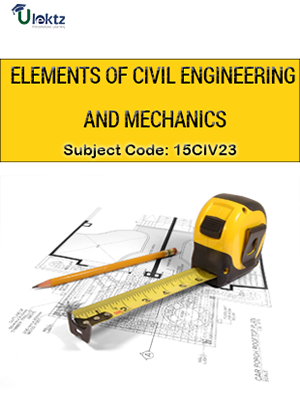uLektz apps

•My WalletMy Order
•My Profile
•My Connections
•My Books
•My Videos
•My Tests
•My Calender
•My Messages
•My Shopping Cart
•My Orders
•Account Settings
•Help

uLektz apps

Book DetailsElements of Civil Engineering and Mechanics

Course Code:15CIV23

Author:uLektz

Regulation:2015

Categories:Civil

Format :ePUB3 (DRM Protected)

Type :eBook

FREE

Description :Elements of Civil Engineering and Mechanics of 15CIV23 covers the latest syllabus prescribed by Visvesvaraya Technological University, Karnataka (VTU) for regulation 2015. Author: uLektz, Published by uLektz Learning Solutions Private Limited.

Note : No printed book. Only ebook. Access eBook using uLektz apps for Android, iOS and Windows Desktop PC.

Topics
Module 1: Introduction to Civil Engineering &Engineering Mechanics

1.1 Introduction to Civil Engineering-Scope of different fields of Civil Engineering -Surveying, Building Materials, Construction Technology, Geotechnical Engineering, Structural Engineering, Hydraulics, Water Resources and Irrigation Engineering, Transportation Engineering, Environmental Engineering.

1.2 Infrastructure: Types of infrastructure, Role of Civil Engineer in the Infrastructural Development, Effect of the infrastructural facilities on socio-economic development of a country

1.3 Roads: Classification of Roads and their functions, Comparison of Flexible and Rigid Pavements (Advantages and Limitations)

1.4 Bridges: Types of Bridges and Culverts, RCC, Steel and Composite Bridges

1.5 Dams: Different types of Dams based on Material, Structural behavior and functionality with simple sketches.

1.6 Introduction to Engineering Mechanics:Basic idealizations - Particle, Continuum and Rigid body; Newton's laws of Force and its characteristics, types of forces-Gravity, Lateral and its distribution on surfaces, Classification of force systems, Principle of physical independence, superposition, transmissibility of forces, Introduction to SI units

1.7 Couple, Moment of a couple, Characteristics of couple, Moment of a force, Equivalent force - Couple system; Numerical problems on moment of forces and couples, on equivalent force - couple system.

Module 2: Analysis of Concurrent Force Systems

2.1 Concepts: Resultants and Equilibrium -Composition of forces - Definition of Resultant; Composition of coplanar -concurrent force system, Parallelogram Law of forces, Principle of resolved parts; Numerical problems on composition of coplanar concurrent force systems.

2.2 Equilibrium of forces - Definition of Equilibrant; Conditions of static equilibrium for different force systems, Lami's theorem; Numerical problems on equilibrium of coplanar – concurrent and non-concurrent force systems.

2.3 Application- Static Friction in rigid bodies in contact-Types of friction, Laws of static friction, Limiting friction, Angle of friction, angle of repose; Impending motion on horizontal and inclined planes; Numerical Problems on single and two blocks on inclined planes

Module - 3 Analysis of Non-Concurrent Force Systems

3.1 Concepts: Resultants and Equilibrium Composition of coplanar - non-concurrent force system, Varignon's principle of moments; Numerical problems on composition of coplanar non-concurrent Force system.

3.2 Application-Support Reaction in beams Types of Loads and Supports

3.3 Statically determinate beams - Numerical problems on support reactions for statically determinate beams with Point load (Normal and inclined) and uniformly distributed and uniformly varying loads and Moments

Module 4 Centroids and Moments of Inertia of Engineering Sections:

4.1 Centroids -Introduction to the concept, centroid of line and area, centroid of basic geometrical figures, computing centroid for– T, L, I, Z and full/quadrant circular sections and their built up sections. Numerical problems.

4.2 Moment of Inertia -Introduction to the concept, Radius of gyration, Parallel axis theorem, Perpendicular axis theorem, Moment of Inertia of basic planar figures, computing moment of Inertia for – T, L, I, Z and full/quadrant circular sections and their built up sections. Numerical problems

Module 5: Kinematics

5.1 Concepts and Applications-Definitions – Displacement – Average velocity – Instantaneous velocity – Speed – Acceleration - Average acceleration – Variable acceleration – Acceleration due to gravity – Newton’s Laws of Motion.

5.2 Rectilinear Motion-Numerical problems.

5.3 Curvilinear Motion – Super elevation – Projectile Motion – Relative motion – Numerical problems.

5.4 Motion under gravity- Numerical problems

Related Books